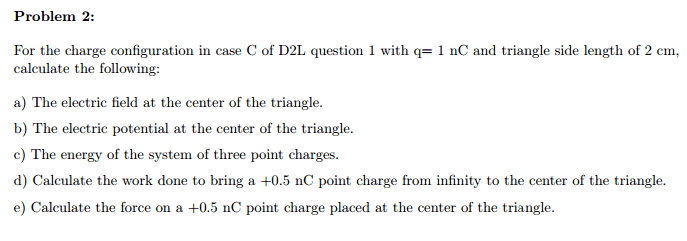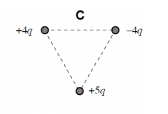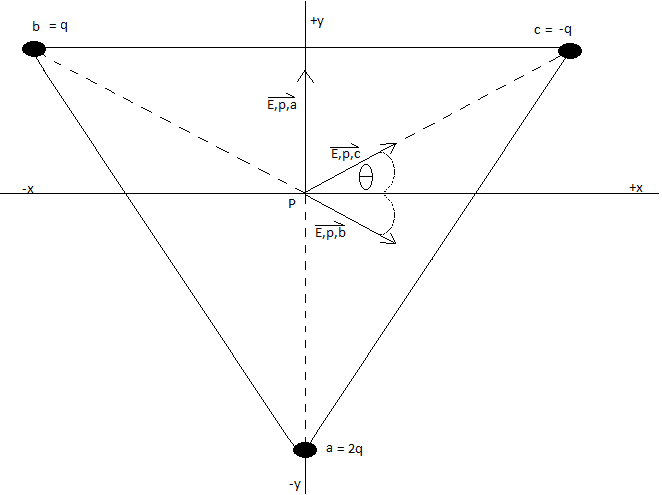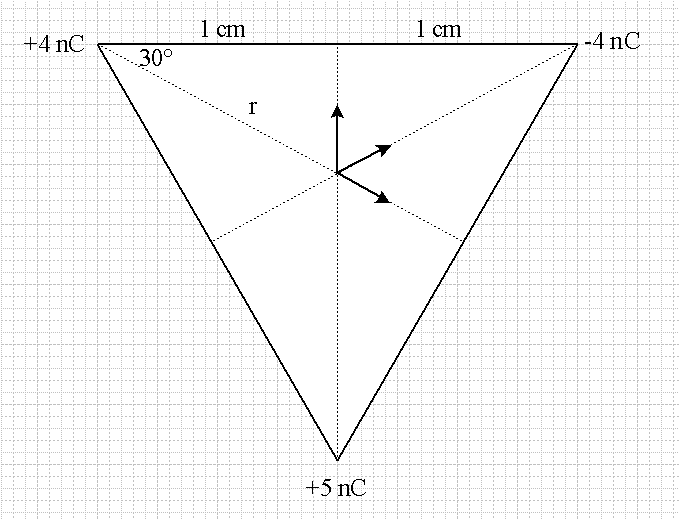# Electric field, potential, work and force

vysero

## Homework Statement[/B]
For the charge configuration in case C of D2L question 1 with q = 1 nC and triangle side length of 2 cm, calculate the following:

a)The electric field at the center of the triangle.
b)The electric potential at the center of the triangle.
c)The energy of the system of three point charges.
d)Calculate the work done to bring a +0.5 nC point charge from infinity to the center of the triangle.
e)Calculate the force on a +0.5 nC point charge placed at the center of the triangle.

## Homework Equations

[/B]
a) E = (kQ)/r^2
b) Ep(tot) = k( (q1/r)+(q2/r) ...)
c)
d) U(tot)=k((q1q2/r)+(q3q4/r)...) and U = -W
e) F = k(abs(q1)abs(q2)/r^2)

## The Attempt at a Solution

[/B]
a) For a I made a force diagram similar to this:I came up with: 412764 n/c x(hat) + 257978 n/c y(hat)

The angle I used for theta was 30* and the distance I came up with for r was .0132 m.

b) For b I calculated that V = 3405.3 volts

c) This question was a bit confusing I made the assumption that by energy my teacher meant for me to take V and divide out the charge but I could definitely be wrong. That being said I came up with a value of 6.8x10^11 Joules, which seems high to me.

d) I am also confused about this question. I am still a bit hazy on the relationship between potential energy and work. What I decided to do was calculate the total potential energy U for the four particle system then I tacked on a negative sign and called it the work... here is the number I came up with: W = -1.7x10^-6 Joules.

e) For e I made a similar force diagram that I made for a. I came up with a total force of:
2.06x10^-4 N x(hat) - 1.3x10^-4 N y(hat)

After finishing this question I feel as though I might be close on a few of the answers but I am still not confident on most of them. Any help would be appreciated, thanks.

Last edited by a moderator:

Mentor
For (a) recheck your calculation for r. How did you determine it?

You'll want to redo (b) if you find a different value for r after checking.

For (c) you're meant to calculate the energy required to assemble the system. that is, the sum of the work done to bring in one charge at a time from infinity. Typically this is done by summing the potential energy of all the charges taken pair-wise.

For (d) you want to move a charge from infinity (potential zero) to the potential at the center of the triangle. So you're moving some charge Q through potential difference V (you already have the potential at the center from (b). The work required is...? This is just adding another charge to the system of (c), but they want the work done (ore resulting PE) for this one charge being moved to its new home.

For (e) you will already have the field in vector form from (a), so what's the force of field E on charge Q?

Homework Helper
Diagram 1 lists charges +4q, -4q, +5q ... but your diagram 2 lists +q, -q, and +2q .
If the side is supposed to be .020m, isn't the half-side 0.010m = r * cos 30 ?
c) total Potential Energy means q3 (V2 + V1) + q2 (V1) , or similar sum
... pretending to put q1 in place first (takes no Work) , then q2 (needs Work against V1), then q3.
V is the Potential, not the Potential Energy ... it already HAS the (moving object) charge divided out.
d) part b already has the Potential V at the center ... the Work to bring q4 to there is just q4 * Vcenter
it doesn't make sense that you know how to calculate U (=PE) for a 4-particle system, but not for a 3-particle system!
using a few nanoCoulomb, your V should be a few kiloVolt, so their PE would be a few microJoule.
e) your E-field at the center has both components positive (probly should with either set of charge values)
but your F vector has a negative component ... how did that get there?

vysero
For (a) recheck your calculation for r. How did you determine it?

You'll want to redo (b) if you find a different value for r after checking.

For (c) you're meant to calculate the energy required to assemble the system. that is, the sum of the work done to bring in one charge at a time from infinity. Typically this is done by summing the potential energy of all the charges taken pair-wise.

For (d) you want to move a charge from infinity (potential zero) to the potential at the center of the triangle. So you're moving some charge Q through potential difference V (you already have the potential at the center from (b). The work required is...? This is just adding another charge to the system of (c), but they want the work done (ore resulting PE) for this one charge being moved to its new home.

For (e) you will already have the field in vector form from (a), so what's the force of field E on charge Q?

Okay I was a bit confused trying to find r. How about .009m rounded up?

Okay for c based on what your saying I should do what I did in e but for 3 particles correct? That's:
-8x10^-16 J
is this correct?

For d, it will be V(5x10^10 C) ?

For e I am a bit confused on how to relate my vector form of E from a. E is in n/c so can I just multiply by the charge? Like say its: (5(n/c) x(hat) + 4(n/c)y(hat))(total charge)?

Homework Helper
yes, the E-field is simpler to calculate ... and add ... then you just multiply by q4 when you're done.
Same for Potential, to yield PE .
I'm not sure what your charges are supposed to be, but a 2cm side would have a half-side being 1cm (so r is not 1cm)

vysero
yes, the E-field is simpler to calculate ... and add ... then you just multiply by q4 when you're done.
Same for Potential, to yield PE .
I'm not sure what your charges are supposed to be, but a 2cm side would have a half-side being 1cm (so r is not 1cm)

Sorry by .009 m rounded up I meant I rounded up and converted to get .009 m

Mentor
I'd suggest that you not round intermediate values that you're going to do further calculations with. Otherwise rounding errors will creep into your significant digits. Express the values in the same units that you were given. Here it's centimeters.

Show your calculation for r. I'm finding a value that's just over 1 cm.

Since the value of r will affect every calculation in the problem, it's hard to comment on purely numerical results. You need to show your method to get feedback on whether what you're doing is correct or not.

vysero
So my method for finding r:

I split the equilateral triangle in half. That gives me a right triangle with a hyp of 2cm or .02m and an adj side of 1cm or .01m. I then used pyth. theorem like:
(.01)^2 +?^2 = (.02)^2 ==> ? = ((.02)^2 - (.01)^2)^(1/2) = .0173 m for the opp side. To find r I said r would be .0173/2 = .00865 which I rounded to .009.

Mentor
So my method for finding r:

I split the equilateral triangle in half. That gives me a right triangle with a hyp of 2cm or .02m and an adj side of 1cm or .01m. I then used pyth. theorem like:
(.01)^2 +?^2 = (.02)^2 ==> ? = ((.02)^2 - (.01)^2)^(1/2) = .0173 m for the opp side. To find r I said r would be .0173/2 = .00865 which I rounded to .009.
Ah. Well there's a problem with that method. The distances from the vertices to that "center" would not all be the same. In fact, you'd find a different "center" for each vertex.

The center of an equilateral triangle is found by finding the intersection of the lines that bisect each vertex angle. That is, draw a line segment from each vertex to the center point of the side opposite. Where these lines intersect is the center:vysero
Ah. Well there's a problem with that method. The distances from the vertices to that "center" would not all be the same. In fact, you'd find a different "center" for each vertex.

The center of an equilateral triangle is found by finding the intersection of the lines that bisect each vertex angle. That is, draw a line segment from each vertex to the center point of the side opposite. Where these lines intersect is the center:

View attachment 78957

Oh I see now okay so I come up with 1.155 cm or .012m if I round up. Is that correct?

Mentor
Oh I see now okay so I come up with 1.155 cm or .012m if I round up. Is that correct?
Muuuuch better :)

vysero
Muuuuch better :)

Great I just have one more question (thanks for your help btw). For part e what I did was take my E vector and I multiplyied it by the charge being brought in from infinity like:

(432531.6 (n/c) x(hat) + 312152.8 (n/c) y(hat))(5x10^-10 C) = 2.2x10^-4 N x(hat) + 1.6x10^-4 N y(hat)

Was this correct or do I need to multiply by the total charge?

Mentor
You want the force on that charge due to the electric field present, so you use only that charge are the field. The charge "doesn't care" how that field came to be, so the other charges are irrelevant at that point.